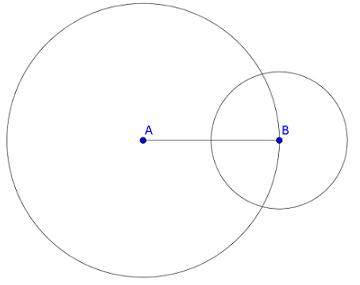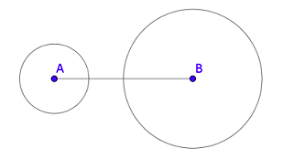# Geometry

## Objective

Determine if three side lengths will create a unique triangle or no triangle.

## Common Core Standards

### Core Standards

?

• 7.G.A.2 — Draw (freehand, with ruler and protractor, and with technology) geometric shapes with given conditions. Focus on constructing triangles from three measures of angles or sides, noticing when the conditions determine a unique triangle, more than one triangle, or no triangle.

## Criteria for Success

?

1. Investigate creating triangles with different side lengths.
2. Understand why a triangle cannot be created if the sum of two side lengths is less than the third side.
3. Determine if a given set of side lengths will create a triangle.
4. Determine a measure of a third side length, given two other sides of a triangle.

## Tips for Teachers

?

The following materials are needed for this lesson: AngLegs or wooden skewers (see the blog post Triangle Inequality Skewers by Elissa Miller), compasses, rulers.

#### Fishtank Plus

• Problem Set
• Student Handout Editor
• Vocabulary Package

## Anchor Problems

?

### Problem 1

Using AngLegs or wooden skewers, investigate different side lengths that will create a triangle.

Complete the chart below, adding rows as needed.

 Side length $a$ Side length $b$ Side length $c$ Triangle? If not, explain why

What conclusion can you make about the side lengths of a triangle?

### Problem 2

In each diagram below, line segment ${AB}$  is 4 units. Two circles are drawn with a given radius and center at point $A$ and point $B$.

 Diagram 1: Circle with center $A$ has a radius of 4 units. Circle with center $B$ has a radius of 2 units.Diagram 2: Circle with center $A$ has a radius of 1 unit. Circle with center $B$ has a radius of 2 units.1. Using Diagram 1, explain or show why you can create a triangle with side lengths 4 units, 4 units, and 2 units.
2. Using Diagram 2, explain or show why you cannot create a triangle with side lengths 4 units, 1 unit, and 2 units.

## Problem Set

?The following resources include problems and activities aligned to the objective of the lesson that can be used to create your own problem set.

?

### Problem 1

Which of the following set of side lengths could not be used to create a triangle?

1. 2 cm, 6 cm, 7 cm
2. 3 cm, 3 cm, 3 cm
3. 4 cm, 5 cm, 8 cm
4. 5 cm, 7 cm, 12 cm

### Problem 2

A triangle has two side lengths that measure 6 in. and 9 in. Which of the following could be the measure of the third side? Select all that apply.

1.   3
2.   4
3.   8
4.   11
5.   14
6.   15
7.   18

?